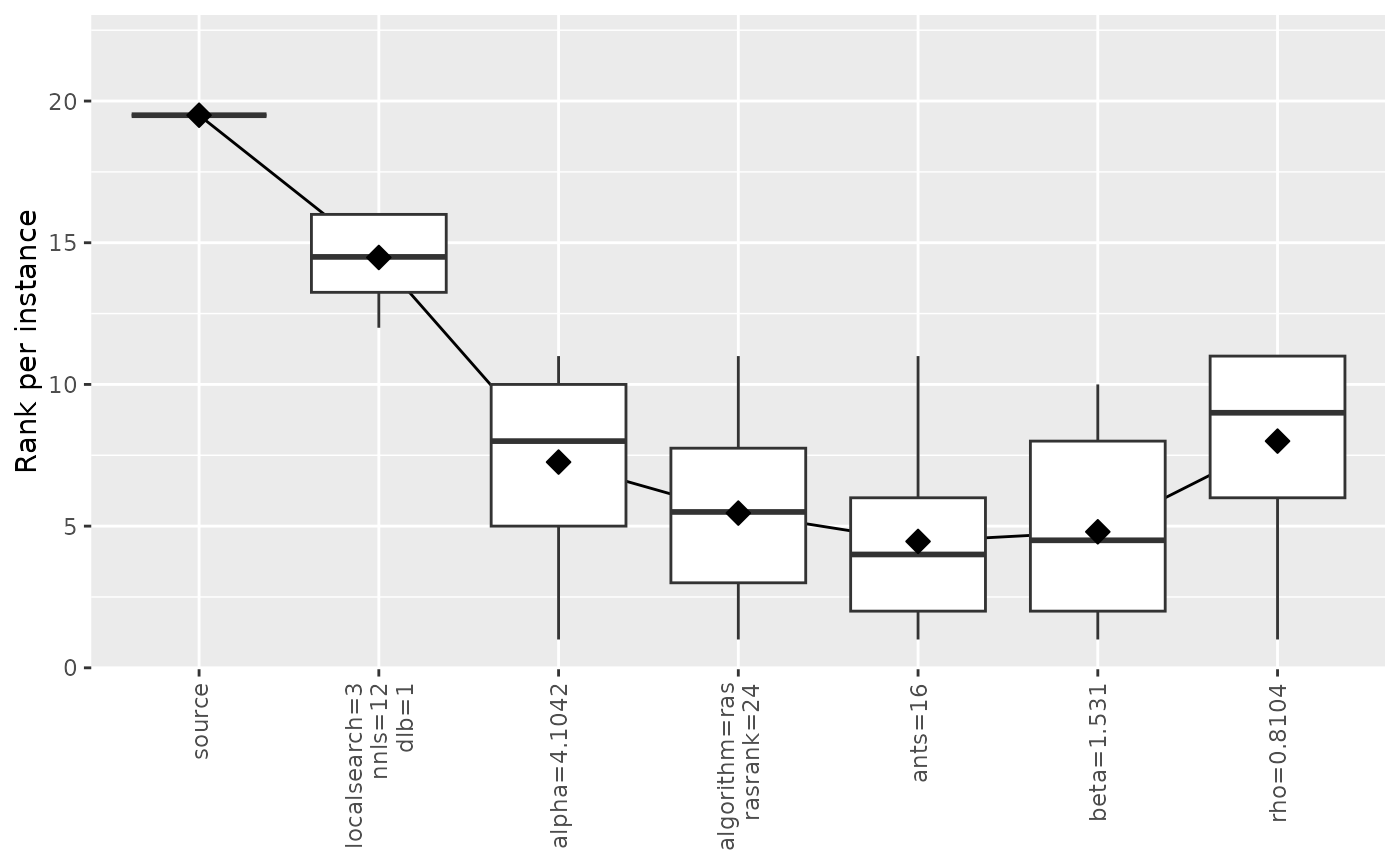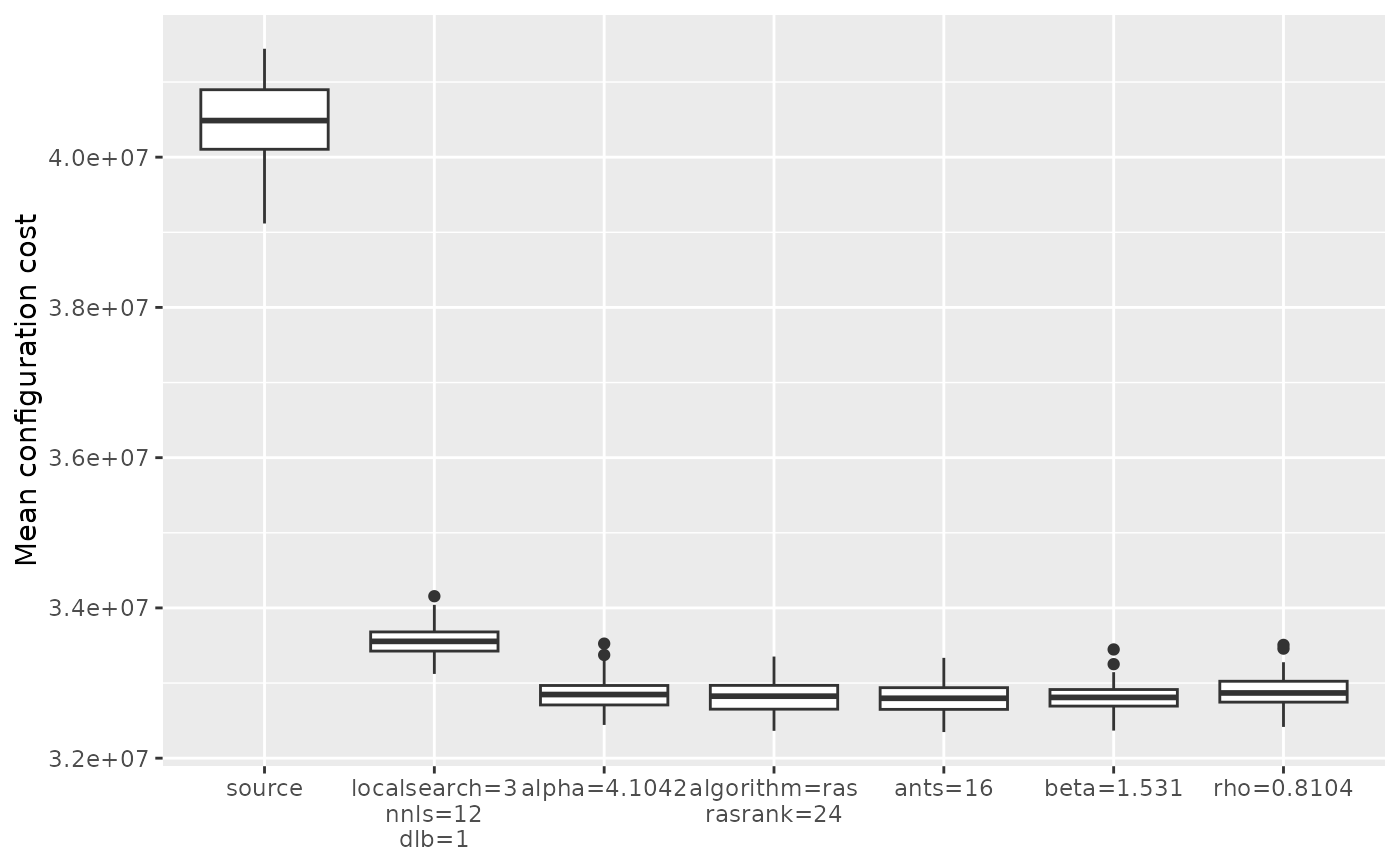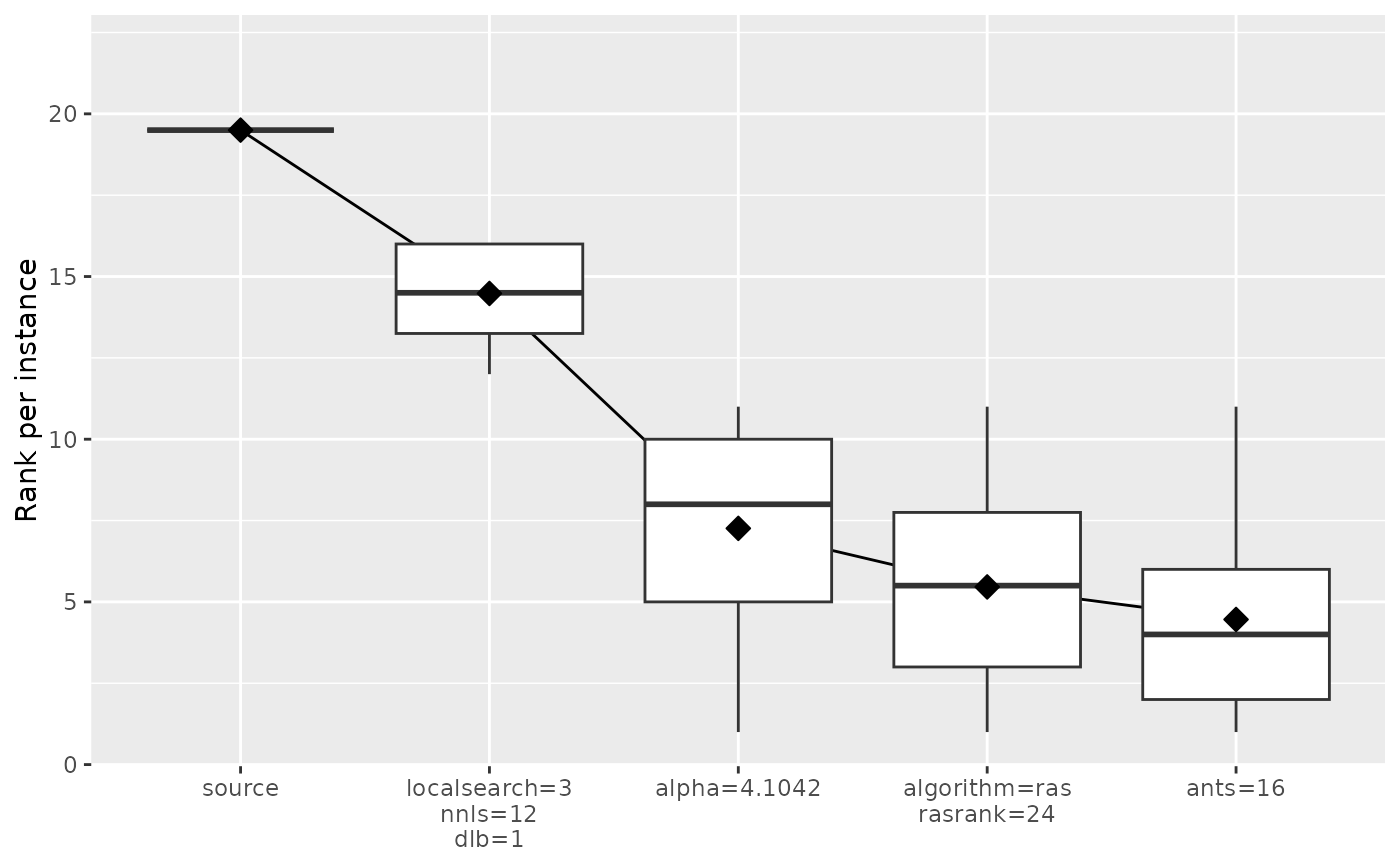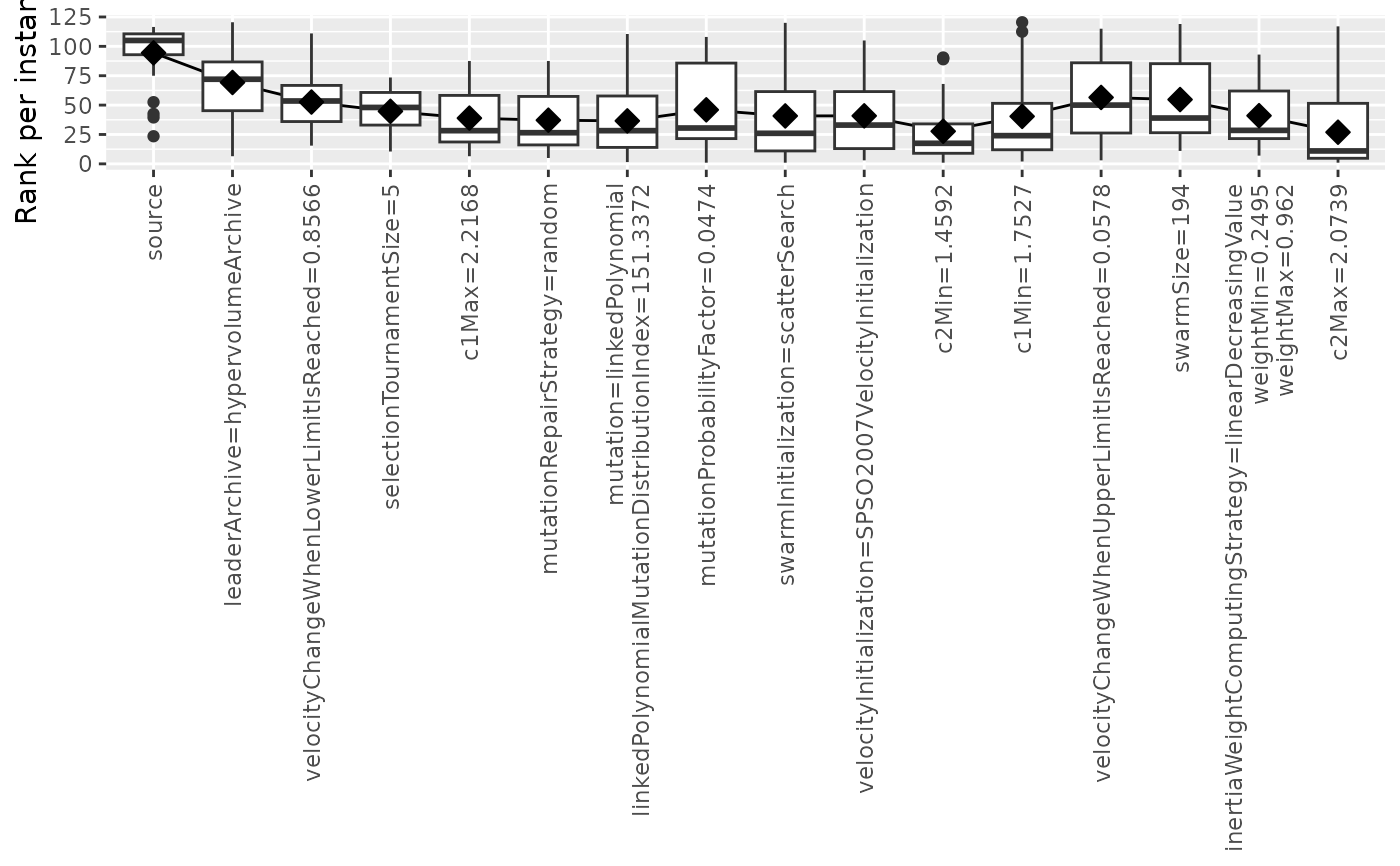Create plot from an ablation log

ablation_plot(
ablog,
type = c("mean", "boxplot", "rank"),
n = 0L,
ylab = "Mean configuration cost",
ylim = NULL,
rotate_labs = TRUE,
filename = NULL
)

## Arguments

ablog

(list()|character(1)) Ablation log object returned by irace::ablation(). Alternatively, the path to an .Rdata file, e.g., "log-ablation.Rdata", from which the object will be loaded.

type

Type of plot. Supported values are "mean" and "boxplot". Adding "rank" will plot rank per instance instead of raw cost value.

n

(integer(1)) Number of steps included in the plot. By default all steps from source to target are included.

ylab

Label of y-axis.

ylim

Numeric vector of length 2 giving the y-axis range.

rotate_labs

(logical(1)) Whether to rotate labels in x-axis. They are rotated by default because they are typically large.

filename

(character(1)) File name to save the plot, for example "~/path/example/filename.png".

## Value

ggplot2::ggplot() boxplot object

irace::ablation(), irace::plotAblation()

## Author

Manuel López-Ibáñez

## Examples

ablog <- read_ablogfile(system.file(package="irace", "exdata", "log-ablation.Rdata"))
ablation_plot(ablog)ablation_plot(ablog, type="boxplot", rotate_labs = FALSE)ablation_plot(ablog, type="mean,boxplot", rotate_labs = FALSE)ablation_plot(ablog, type="rank,mean,boxplot", n = 4, rotate_labs = FALSE)ablog <- system.file(package="iraceplot", "exdata", "log-ablation-autoMOPSODTLZ.Rdata")
ablation_plot(ablog, type="rank,mean,boxplot")